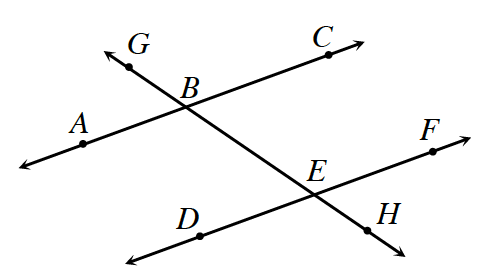### Home > CCG > Chapter 9 > Lesson 9.1.5 > Problem9-63

9-63.

Multiple Choice: For $∠ABE ≅ ∠BEF$ in the diagram below, what must be true?

1. $∠ABE ≅ ∠BED$

2. $∠ABE ≅ ∠GBC$

3. $\overline{AC}//\overline{GH}$

4. $\overline{AC}//\overline{DF}$

5. None of theseThe given angles are alternate interior angles. What does this tell you?
Also, there is more than one correct answer in this case.

b and d